CLAT  >  Test: Quantitative Techniques- 2

# Test: Quantitative Techniques- 2 - CLAT

Test Description

## 20 Questions MCQ Test Mock Test Series for CLAT - Test: Quantitative Techniques- 2

Test: Quantitative Techniques- 2 for CLAT 2023 is part of Mock Test Series for CLAT preparation. The Test: Quantitative Techniques- 2 questions and answers have been prepared according to the CLAT exam syllabus.The Test: Quantitative Techniques- 2 MCQs are made for CLAT 2023 Exam. Find important definitions, questions, notes, meanings, examples, exercises, MCQs and online tests for Test: Quantitative Techniques- 2 below.
Solutions of Test: Quantitative Techniques- 2 questions in English are available as part of our Mock Test Series for CLAT for CLAT & Test: Quantitative Techniques- 2 solutions in Hindi for Mock Test Series for CLAT course. Download more important topics, notes, lectures and mock test series for CLAT Exam by signing up for free. Attempt Test: Quantitative Techniques- 2 | 20 questions in 30 minutes | Mock test for CLAT preparation | Free important questions MCQ to study Mock Test Series for CLAT for CLAT Exam | Download free PDF with solutions
 1 Crore+ students have signed up on EduRev. Have you?
Test: Quantitative Techniques- 2 - Question 1

### In a class average age of 24 boys and their teacher is 15 years. When the teacher's age is excluded the average age decreases by one year. The age of the teacher is?

Detailed Solution for Test: Quantitative Techniques- 2 - Question 1

Sum of the ages of 24 boys and 1 teacher = (15 x 25) years = 375 years.
Sum of the ages of 24 boys = (24 x 14) years = 336 years.
Age of the teacher = (375 − 336) years = 39 years.

Test: Quantitative Techniques- 2 - Question 2

### The monthly income of P and Q are in ratio 2:3 and their monthly expenses are in ratio 5:9. If each of them save 300 per month, their monthly income is:

Detailed Solution for Test: Quantitative Techniques- 2 - Question 2

Income of A = 2x
Income of B = 3x

Savings of A  =  300
Savings of B  =  300

Monthly expense are in ratio 5:9
(2x-300) / (3x-300) =  5/9
⇒ 18x - 2700 = 15x - 1500
⇒ 3x = 1200
⇒ x =  400

Therefore,
Income of A = 2x = 2 * 400 = 800
Income of B = 3x = 3 * 400 = 1200

Test: Quantitative Techniques- 2 - Question 3

### Man covers a certain distance by driving a car at the speed of 60 km/h and return back to the starting point at the speed of 40 km/h. Find its average speed?

Detailed Solution for Test: Quantitative Techniques- 2 - Question 3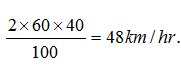Test: Quantitative Techniques- 2 - Question 4

Two numbers are such that the ratio between them is 3:4 but if each is increased by 3 then the ratio becomes 4:5. Find the difference between the numbers?

Detailed Solution for Test: Quantitative Techniques- 2 - Question 4

Let the two numbers be 3x, 4x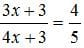⇒ 15x + 15 = 16x + 12
⇒ x = 3
Diff = (4*3) - (3*3) = 3

Test: Quantitative Techniques- 2 - Question 5

At present, the age of the father is five times that the age of the son. Three years hence, the father's age would be four times that of his son. Find the present age of the father and the son?

Detailed Solution for Test: Quantitative Techniques- 2 - Question 5

Let the son's age be x.
⇒ Father's age = 5x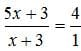⇒ 5x + 3 = 4x + 12
⇒ x = 9
Hence, son's age is 9 and father's age is 45.

Test: Quantitative Techniques- 2 - Question 6

If 25% of a number is 65, then 45% of the number will be?

Detailed Solution for Test: Quantitative Techniques- 2 - Question 6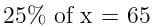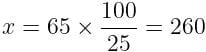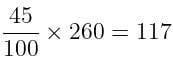Test: Quantitative Techniques- 2 - Question 7

A and B have money in the ratio 2:1. If A gives Rs. 2 to B, the money will be in the ratio 1:1. What were the initial amounts they had?

Detailed Solution for Test: Quantitative Techniques- 2 - Question 7

Before lending the money, the ratio of money A and B = 2:1
After lending the money, the ratio of money A and B = 1:1
Since, A gives B two rupees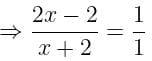⇒ 2x - 2 = x + 2
⇒ x = 4

A = 2 × 4 = 8, B = 1 × 4 = 4

Test: Quantitative Techniques- 2 - Question 8

18 men can reap a field in 50 days. In how many days will 30 men can complete the same work?

Detailed Solution for Test: Quantitative Techniques- 2 - Question 8

18 * 50 = Days * 30
⇒ Days = 30

Test: Quantitative Techniques- 2 - Question 9

A is working and B is sleeping partner in a firm. A invest Rs 2100 and B invests Rs 3100. A being working partner gets 25% of the profits, and the balance of the profits to be divided in the proportion to their capitals. What did each get out of profit of Rs 1040?

Detailed Solution for Test: Quantitative Techniques- 2 - Question 9

Total profit = 1040.
A's 25% profit = 1040 * 1/4 = 260
Remaining profit = 780
Ratio of investment of A and B = 2100:3100 = 21:31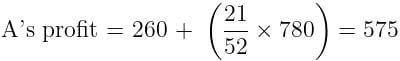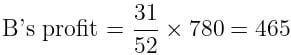Test: Quantitative Techniques- 2 - Question 10

A man has some hens and cows. If the number of heads be 48 and the number of feet equals 140, then the number of hens will be:

Detailed Solution for Test: Quantitative Techniques- 2 - Question 10

Let the number of hens be x and the number of cows be y.
Then, x + y = 48 .... (i)
and 2x + 4y = 140
⇒  x + 2y = 70 .... (ii)
Solving (i) and (ii) we get: x = 26, y = 22.
∴ The required answer = 26.

Test: Quantitative Techniques- 2 - Question 11

If a man can row at the rate of 5kmph instill water and his rate against current is 3.5kmph, then man's rate of speed along current is?

Detailed Solution for Test: Quantitative Techniques- 2 - Question 11

Speed of Boat = 5 Km/h
Speed upstream = 3.5 km/h

Speed of upstream = Speed of boat - Speed of water

⇒ Speed of water = 5 - 3.5 = 1.5 km/h
⇒ Speed downstream = 5 + 1.5 = 6.5 km/h

Test: Quantitative Techniques- 2 - Question 12

If a square field has its area equal to 324m2 then the perimeter of the field?

Detailed Solution for Test: Quantitative Techniques- 2 - Question 12

Area = 342 m2
⇒ Side = √342 = 18m
⇒ Perimeter = 18 x 4 = 72m

Test: Quantitative Techniques- 2 - Question 13

X alone can complete a piece of work in 40 days. He worked for 8 days and left. Y alone complete the remaining work in 16 days. How long would X and Y together take to complete the work?

Detailed Solution for Test: Quantitative Techniques- 2 - Question 13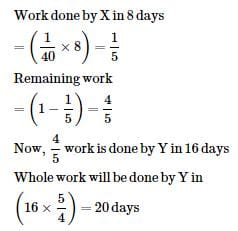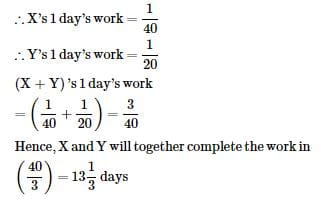Test: Quantitative Techniques- 2 - Question 14

The difference between simple interest and compound interest on certain sum of money for 2 years 4% per annum is Rs 1. Find the sum?

Detailed Solution for Test: Quantitative Techniques- 2 - Question 14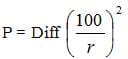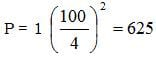Test: Quantitative Techniques- 2 - Question 15

By selling a bicycle for Rs. 2,850, a shopkeeper gains 14%. If the profit is reduced to 8%, then the selling price will be:

Detailed Solution for Test: Quantitative Techniques- 2 - Question 15

Let Cost Price was X.
X + 14% of X = 2850
X + 0.14X = 2850
1.14X = 2850
X = 2500.
⇒ Cost Price = Rs. 2500.
Now, Selling Price when profit remains at 8% = 2500 + 8% of 2500 = Rs. 2700

Test: Quantitative Techniques- 2 - Question 16

A men went to RBI with Rs 2000. He asked the cashier to give him Rs 10 and Rs 20 notes only in return. The man got 150 notes in all. How many Rs 10 notes did he get in all?

Detailed Solution for Test: Quantitative Techniques- 2 - Question 16

x + y = 150 ........(i)
10x + 20y = 2000
⇒  x + 2y = 200 .......(ii)
Solving (i) and (ii),we get
y = 50, x = 100

Test: Quantitative Techniques- 2 - Question 17

If price of a book is first decreased by 25% and then increased by 20% the net percentage change in the price of the book will be

Detailed Solution for Test: Quantitative Techniques- 2 - Question 17

Let the original price be Rs. 100.

New final price = 120% of (75% of Rs. 100) = 0.12 * (0.75 * 100) = Rs. 90.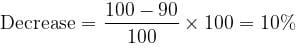Test: Quantitative Techniques- 2 - Question 18

A dice is rolled twice. What is probability of getting the sum 5?

Detailed Solution for Test: Quantitative Techniques- 2 - Question 18

Sum of 5 is obtained as (1,4), (2,3), (3,2), (4,1)
⇒ Probability = 4/36 = 1/9

Test: Quantitative Techniques- 2 - Question 19

12345 + 13000 + 41240 + 8 = ?

Detailed Solution for Test: Quantitative Techniques- 2 - Question 19

12345 + 13000 + 41240 + 8 = 66593

Test: Quantitative Techniques- 2 - Question 20

Three numbers are in the ratio  1 : 2 : 3  and their H.C.F is 12. The numbers are

Detailed Solution for Test: Quantitative Techniques- 2 - Question 20

Let the required numbers be x, 2x, 3x.
Since H.C.F. = 12
⇒ The numbers are: 12, 24, 36

## Mock Test Series for CLAT

2 videos|10 docs|83 tests
Information about Test: Quantitative Techniques- 2 Page
In this test you can find the Exam questions for Test: Quantitative Techniques- 2 solved & explained in the simplest way possible. Besides giving Questions and answers for Test: Quantitative Techniques- 2, EduRev gives you an ample number of Online tests for practice

## Mock Test Series for CLAT

2 videos|10 docs|83 tests